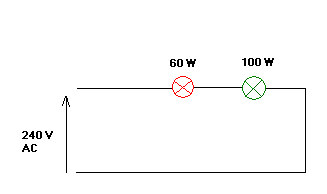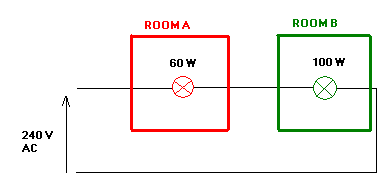Home » Topics » English riddles » General Subjects » Electrical riddle no. 8 – Lamp glow

# Electrical riddle no. 8 – Lamp glow

• This topic has 5 replies, 1 voice, and was last updated 2 years, 1 month ago bymaerskbhoy.
• Creator
Topic
• #61Ratnagiri Ramkumar from INDIA

Two lamps each of 60W & 100W with similar rated voltage are connected in series to a single phase & neutral ,240 V electric supply as per diagram.

Which will glow brighter and why?show the calculation involved?Viewing 5 replies - 1 through 5 (of 5 total)
• Author
Replies
• #1198Hamid

The lamp 60W will be brighter than 100W; because: R60W > R100W & (P60W = R60W.I2 ) > (P100W = R100W.I2)But let us assume the lamp 60w is installed in room A and another lamp is located in room B with same electrical circuit. For maximum lighting achievement in room B, which lamp (rated power) shall be permuted with lamp 100w?

#1262balaji

Replace the 100W lamp with a 60 W lamp

#1274Bill

i have done this before and the 100 watt bulb will burn out with 240 v breaking the circuit befor burning out the 60 watt

#1288doorbar

(a)The question is rather misleading. It can be interpreted in two different scenarios: (i) A 240V circuit supplying two lamps expending 60W and 100W power respectively. In this case, the 100W lamp will be brighter with a resistance =11.875?, 60W with R=7.125?. (ii) Two 240V lamps rated at 60W and 100W respectively are connected in series. In this case, the result will be as Hamid has given. Both rooms will have a 15W lighting outpu, total for two rooms is 30W. (b) Answer to Hamid’s question, based on (ii) above, use maximum power transfer principle, assume the source is an ideal source, add a 150W in series with 100W in room B. (c) Bill, the bulbs will not burn out unless they were at a much lower voltage rating (i.e., 50V rated). Since they are connected in series, they cannot burn out separately. They will need to be burnt out simultaneously.

#1385maerskbhoy

first of all, given is so vague that complete rating of the bulb was not mentioned. it is essential that voltage rating ( or current) of the bulb be clearly stated. for the sake of discussion, let us say that each bulb are rated 60W and 100W and 240V each.. calculating the rated resistance of each lamp using P = V2/R, we can calculate the resistance of the 60W lamp as 960 ohms and resistance of the 100W lamp as 576 ohms. so when they are connected in series across a 240V source, total resistance would be equal to 1536 ohms giving a current ( I = V/R) of 0.1563 ampere. getting again the power consumed by each lamp when connected in series across a 240V source using I2R formula, the power across 60W lamp will now be 23.42 watts only while the power on the 100W lamp is 14.05 watts only. considering the setup, the 60W lamp will glow brighter than the 100W lamp. it would arrive into a different answer when configurations where known and altered like: 1. bulbs are rated 120V only 2. 1st bulb is 120V and another bulb is 240V 3. or author is thinking of a given like 60? and 100? rather than 60W and 100W where in this case the 100? bulb will consume 225Watts while 60? is 135 watts only, thus 100? is brighter. ————————- YEBAH…..

Viewing 5 replies - 1 through 5 (of 5 total)
• You must be logged in to reply to this topic.